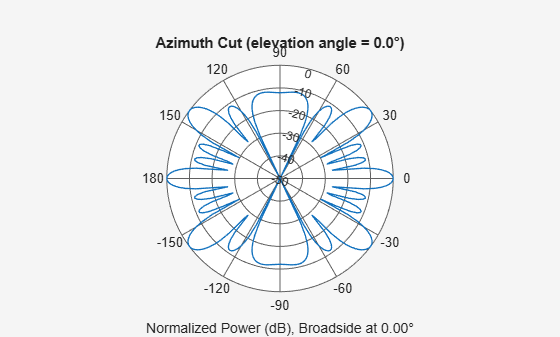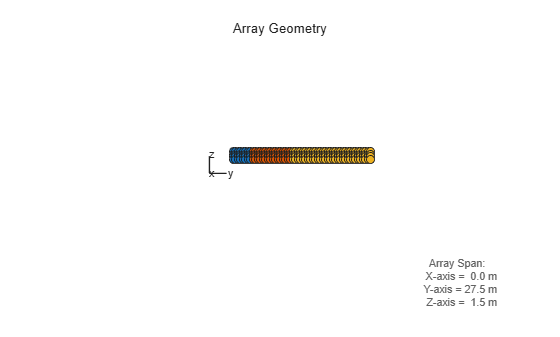# phased.PartitionedArray

Phased array partitioned into subarrays

## Description

The `PartitionedArray` object represents a phased array that is partitioned into one or more subarrays.

To obtain the response of the subarrays in a partitioned array:

1. Define and set up your partitioned array. See Construction.

2. Call `step` to compute the response of the subarrays according to the properties of `phased.PartitionedArray`. The behavior of `step` is specific to each object in the toolbox.

You can also specify a `PartitionedArray` object as the value of the `SensorArray` or `Sensor` property of objects that perform beamforming, steering, and other operations.

### Note

Starting in R2016b, instead of using the `step` method to perform the operation defined by the System object™, you can call the object with arguments, as if it were a function. For example, `y = step(obj,x)` and `y = obj(x)` perform equivalent operations.

## Construction

`H = phased.PartitionedArray` creates a partitioned array System object, `H`. This object represents an array that is partitioned into subarrays.

`H = phased.PartitionedArray(Name,Value)` creates a partitioned array object, `H`, with each specified property Name set to the specified Value. You can specify additional name-value pair arguments in any order as (`Name1`,`Value1`,...,`NameN`,`ValueN`).

## Properties

 `Array` Sensor array Sensor array, specified as any array System object belonging to Phased Array System Toolbox™. Default: `phased.ULA('NumElements',4)` `SubarraySelection` Subarray definition matrix Specify the subarray selection as an M-by-N matrix. M is the number of subarrays and N is the number of elements in the array. Each row of the matrix corresponds to a subarray and each entry in the row indicates whether or not an element belongs to the subarray. When the entry is zero, the element does not belong to the subarray. A nonzero entry represents a complex-valued weight applied to the corresponding element. Each row must contain at least one nonzero entry. The phase center of each subarray is at the subarray geometric center. The `SubarraySelection` and `Array` properties determine the geometric center. Default: `[1 1 0 0; 0 0 1 1]` `SubarraySteering` Subarray steering method Specify the method of subarray steering as either `'None'` | `'Phase'` | `'Time'` | `'Custom'`. When you set this property to `'Phase'`, a phase shifter is used to steer the subarray. Use the `STEERANG` argument of the `step` method to define the steering direction.When you set this property to `'Time'`, subarrays are steered using time delays. Use the `STEERANG` argument of the `step` method to define the steering direction.When you set this property to `'Custom'`, subarrays are steered by setting independent weights for all elements in each subarray. Use the `WS` argument of the `step` method to define the weights for all subarrays. Default: `'None'` `PhaseShifterFrequency` Subarray phase shifter frequency Specify the operating frequency of phase shifters that perform subarray steering. The property value is a positive scalar in hertz. This property applies when you set the `SubarraySteering` property to `'Phase'`. Default: `300e6` `NumPhaseShifterBits` Number of phase shifter quantization bits The number of bits used to quantize the phase shift component of beamformer or steering vector weights. Specify the number of bits as a non-negative integer. A value of zero indicates that no quantization is performed. Default: `0`

## Methods

 collectPlaneWave Simulate received plane waves directivity Directivity of partitioned array getElementPosition Positions of array elements getNumElements Number of elements in array getNumSubarrays Number of subarrays in array getSubarrayPosition Positions of subarrays in array isPolarizationCapable Polarization capability pattern Plot partitioned array directivity, field, and power patterns patternAzimuth Plot partitioned array directivity or pattern versus azimuth patternElevation Plot partitioned array directivity or pattern versus elevation plotResponse Plot response pattern of array step Output responses of subarrays viewArray View array geometry
Common to All System Objects
`release`

Allow System object property value changes

## Examples

collapse all

Plot the azimuth response of a 4-element ULA partitioned into two 2-element ULA's. The element spacing is one-half wavelength.

Create the ULA, and partition it into two 2-element ULA's.

```sULA = phased.ULA('NumElements',4,'ElementSpacing',0.5); sPA = phased.PartitionedArray('Array',sULA,... 'SubarraySelection',[1 1 0 0;0 0 1 1]);```

Plot the azimuth response of the array. Assume the operating frequency is 1 GHz and the propagation speed is the speed of light.

```fc = 1e9; pattern(sPA,fc,[-180:180],0,'Type','powerdb',... 'CoordinateSystem','polar',... 'Normalize',true)```Create a 4-element ULA. Then partition the ULA into two 2-element ULAs. Then, calculate the response at boresight of a 4-element ULA partitioned into two 2-element ULAs.

```sULA = phased.ULA('NumElements',4,'ElementSpacing',0.5); sPA = phased.PartitionedArray('Array',sULA,... 'SubarraySelection',[1 1 0 0;0 0 1 1]);```

Calculate the response at 1 GHz. The signal propagation speed is the speed of light.

```fc = 1e9; resp = step(sPA,fc,[0;0],physconst('LightSpeed'))```
```resp = 2×1 2 2 ```

Create a partitioned URA array with three subarrays of different sizes. The subarrays have 8, 16, and 32 elements. Use different sets of subarray element weights for each subarray.

Create a 4-by-56 element URA.

```antenna = phased.IsotropicAntennaElement; fc = 300e6; c = physconst('LightSpeed'); lambda = c/fc; n1 = 2^3; n2 = 2^4; n3 = 2^5; nrows = 4; ncols = n1 + n2 + n3; array = phased.URA('Element',antenna,'Size',[nrows,ncols]);```

Select the three subarrays by setting the selection matrix.

```sel1 = zeros(nrows,ncols); sel2 = sel1; sel3 = sel1; sel = zeros(3,nrows*ncols); for r = 1:nrows sel1(r,1:n1) = 1; sel2(r,(n1+1):(n1+n2)) = 1; sel3(r,((n1+n2)+1):ncols) = 1; end sel(1,:) = sel1(:); sel(2,:) = sel2(:); sel(3,:) = sel3(:);```

Create the partitioned array.

```partarray = phased.PartitionedArray('Array',array, ... 'SubarraySelection',sel,'SubarraySteering','Custom'); viewArray(partarray,'ShowSubarray','All');```Set weights for each subarray and get the response of each subarray. Put the weights in a cell array.

```wts1 = ones(nrows*n1,1); wts2 = 1.5*ones(nrows*n2,1); wts3 = 3*ones(nrows*n3,1); resp = partarray(fc,[30;0],c,{wts1,wts2,wts3})```
```resp = 3×1 complex 0.0246 + 0.0000i 0.0738 - 0.0000i 0.2951 - 0.0000i ```

 Van Trees, H.L. Optimum Array Processing. New York: Wiley-Interscience, 2002.

﻿